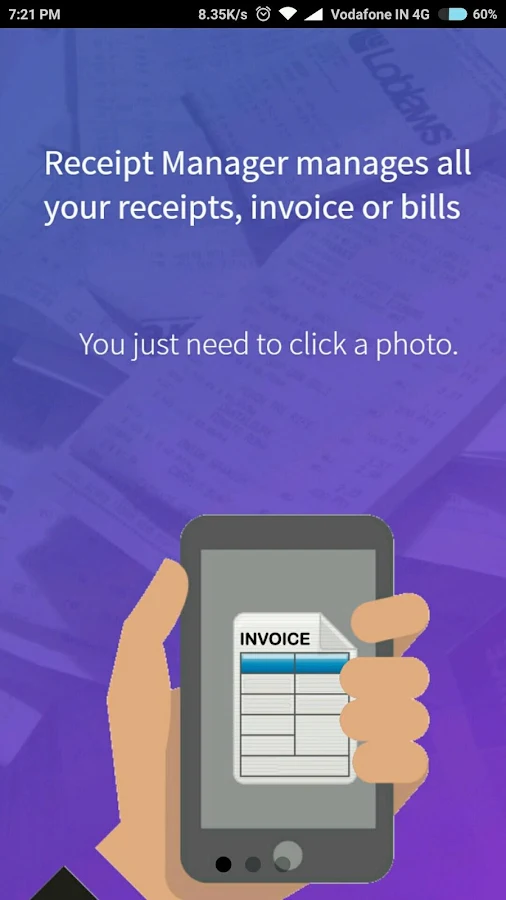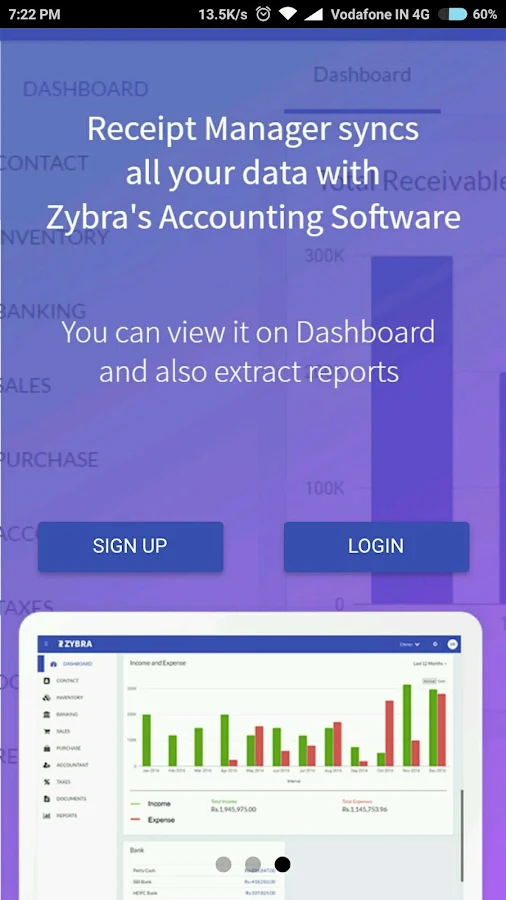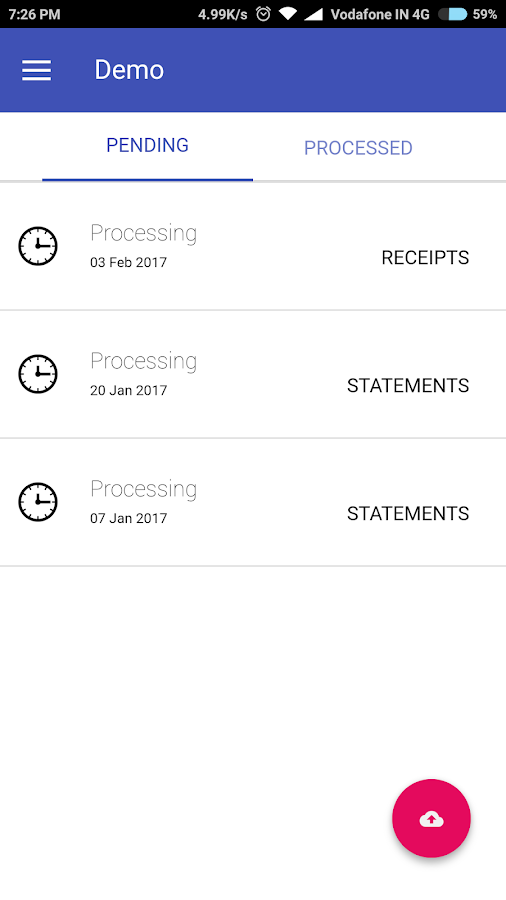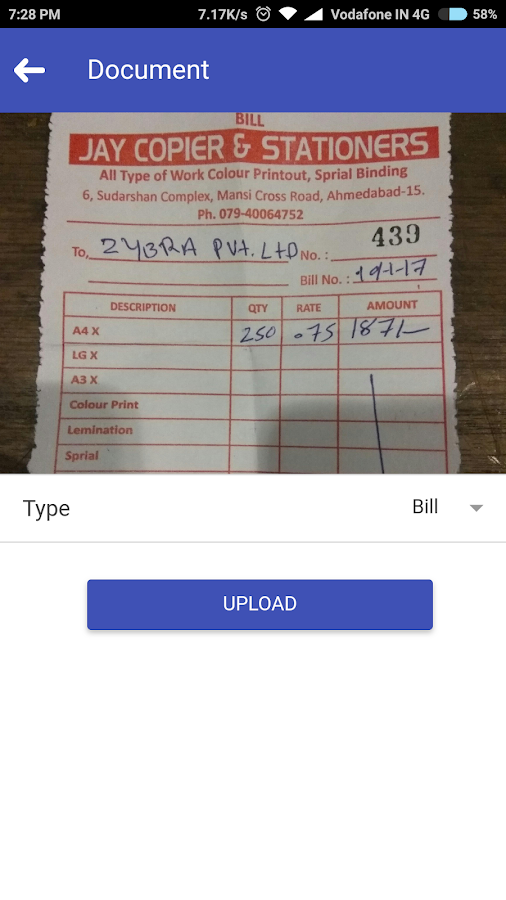# Receipts Manager by ZybraFinance Local
0.0.2
Android 4.1 and up
471k
Full internet access.
5.0 4

## What is Depreciation & How to Calculate Depreciation?

• Posted on Dec 11, 2022
• |
• By Deep Patel

In accounting, depreciation is the process of allocating the cost of a tangible asset over its useful life. This is done by recognizing a portion of the asset’s cost as an expense each year, rather than recognizing the entire cost in the year the asset is acquired. This helps to ensure that the financial statements of a company accurately reflect the value of its assets, and it allows the company to spread the cost of the asset over its useful life. Depreciation is a common accounting practice that is used to reflect the gradual decline in value of an asset over time.

There are several methods for calculating depreciation, each of which is suited to different types of assets and situations. The most common methods are:

1. Straight-line depreciation: This is the simplest and most commonly used method of calculating depreciation. Under this method, the depreciation expense is calculated by dividing the cost of the asset by its expected useful life. For example, if a company purchases a piece of equipment for \$100,000 with an expected useful life of 10 years, the annual depreciation expense would be \$10,000.
2. Declining balance depreciation: This method calculates depreciation by applying a fixed rate to the declining balance of the asset. The fixed rate is typically a multiple of the straight-line depreciation rate, such as double or triple the straight-line rate. For example, if a company purchases a piece of equipment for \$100,000 with an expected useful life of 10 years and a declining balance rate of double the straight-line rate, the first year’s depreciation would be calculated as \$20,000 (2 x \$10,000). In subsequent years, the depreciation expense would be calculated by applying the same rate to the remaining balance of the asset.
3. Units of production depreciation: This method calculates depreciation based on the number of units produced by the asset. This method is typically used for assets that have a variable usage or output, such as a piece of machinery that is used to produce a certain number of products. The depreciation expense is calculated by dividing the cost of the asset by the expected number of units it will produce, and then multiplying that amount by the actual number of units produced.

In general, the calculation of depreciation involves determining the cost of the asset, its expected useful life, and the residual value, and then applying the chosen depreciation method to calculate the annual depreciation expense. The specific steps for calculating depreciation will vary depending on the method used and the specific circumstances of the asset.

In addition to the methods described above, there are several other factors that can affect the calculation of depreciation.

One important factor is the expected useful life of the asset. The useful life of an asset is the period of time over which the asset is expected to be used by the company. This can vary depending on the type of asset, the conditions in which it is used, and the level of maintenance it receives. The expected useful life of an asset is an important input into the calculation of depreciation, as it determines the period over which the cost of the asset will be spread.

Another important factor is the residual value of the asset. The residual value of an asset is the estimated value of the asset at the end of its useful life. This is important because it represents the value of the asset that is not depreciated. For example, if a company purchases a piece of equipment for \$100,000 with an expected useful life of 10 years and a residual value of \$10,000, the total depreciation expense would be \$90,000 over the 10-year period.

In addition to these factors, the method of depreciation used can also affect the calculation of depreciation. As mentioned earlier, there are several methods for calculating depreciation, each of which is suited to different types of assets and situations. For example, the straight-line method is simple and easy to use, but it may not accurately reflect the actual

• Posted on Dec 11, 2022
• |
• By Deep Patel
• |